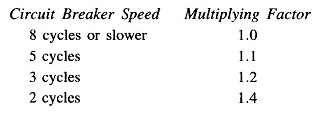## Selection of Circuit Breaker:

There are two types of Selection of Circuit Breaker ratings which require the computation of SC current are: (i) rated momentary current and (ii) rated symmetrical interrupting current.

Symmetrical SC current is obtained by using subtransient reactances for synchronous machines. Momentary current (rms) is then calculated by multiplying the symmetrical momentary current by a factor of 1.6 to account for the presence of DC off-set current.

Symmetrical current to be interrupted is computed by using subtransient reactances for synchronous generators and transient reactances for synchronous motors induction motors are neglected. The DC off-set value to be added to obtain the current to be interrupted is accounted for by multiplying the symmetrical SC current by a factor as tabulated below:If SC MVA (explained below) is more than 500, the above multiplying factors are increased by 0.1 each. The multiplying factor for air breakers rated 600 V or lower is 1.25.

The current that a circuit breaker can interrupt is inversely proportional to the operating voltage over a certain range, i.e.

Amperes at operating voltage = amperes at rated voltage x rated voltage/operating voltage

Of course, operating voltage cannot exceed the maximum design value. Also, no matter how low the voltage is, the rated interrupting current cannot exceed the rated maximum interrupting current. Over this range of voltages, the product of operating voltage and interrupting current is constant. It is therefore logical as well as convenient to express the Selection of Circuit Breaker rating in terms of SC MVA that can be interrupted, defined as

Rated interrupting MVA (three-phase) capacity = √3 |V(line)|rated x |I(line)|rated interrupting current

where

• V(line) is in kV and
• I (line) is kA.

Thus, instead of computing the SC current to be interrupted, we compute three-phase SC MVA to be interrupted, where

SC MVA (3-phase) = √3 x prefault line voltage in kV x SC current in KA.

If voltage and current are in per unit values on a three-phase basis

SC MVA (3-phase) = |V|prefault x |I|SC x (MVA)Base       (9.17)

Obviously, rated MVA interrupting capacity of a circuit breaker is to be more than (or equal to) the SC MVA required to be interrupted.

For the Selection of Circuit Breaker for a particular location, we must find the maximum possible SC MVA to be interrupted with respect to type and location of fault and generating capacity (also synchronous motor load) connected to the system.

A three-phase fault though rare is generally the one which gives the highest SC MVA and a circuit breaker must be capable of interrupting it. An exception is an LG (line-to-ground) fault close to a synchronous generator. In a simple system the fault location which gives the highest SC MVA may be obvious but in a large system various possible locations must be tried out to obtain the highest SC MVA requiring repeated SC computations.

Scroll to Top